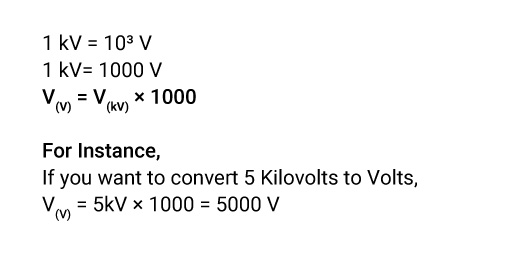## About Our kV to V Converter

“Our converter to Math problems: Keep supplying your problems. I’m here to fix it.”

Gone are the days when you had to spend so much time calculating for small chunks of conversions. But from now onwards, you can use our converter for any metric conversions to save you time.

If you want to convert kilovolts to volts, volts to kilovolts, or another metric conversion, access our tool to get instant solutions.

### Definition of Kilovolts.

A kilovolt is a multiple unit of electrical potential difference or an electromotive force equal to one thousand volts. It is generated when a particle with one charged electron passes through the potential difference of 1,000 Volts in a vacuum.

### What is the Symbol of Kilovolts?

kV is the symbol of Kilovolts.

### How to Convert Kilovolts to Volts?

To convert kilovolts to volts, multiply the Voltage by the conversion ratio. The Voltage in volts equals the kilovolts multiplied by one thousand. One kilovolt is equal to one thousand volts.

### The Kilovolts to Volts Conversion Formula.

Here is the direct formula for kilovolts to volts conversion,If you find this confusing, you can use our kilovolts to volts calculator to get the answer in a short stretch of a second.

### How to Use Our Kilovolts to Volts Converter?

We believe in simplifying your problems, so we have introduced a kilovolts-to-volt converter to get you an instant answer. Know how.

• Step2: Introduce the value you want to convert

### How Many Kilovolts Are in a Volt?

1 V = 10-3 kV

1V = 0.001 kV

One volt is equal to one-thousandth of a kilovolt.

### What is the Difference Between Kilovolts and Volts?

• Kilovolt is a multiple of volt and is often used to measure large quantities in Voltage. kV is the symbol of Kilovolts. One kilovolt consists of one thousand volts.
• Volt is the SI unit of Voltage, and V is the symbol of Voltage. One volt equals one-thousandth of a kilovolt.

### What is the Kilovolts Unit of Measure?

Kilovolt (kV) is the unit of electromotive force equal to 1000 Volts.

### Examples of Converting Kilovolts to Volts.

V(V) = 3kV × 1000 = 3000 V

V(V) = 6kV × 1000 = 6000 V

V(V) = 7kV × 1000 = 7000 V

V(V) = 10kV × 1000 = 10000 V

### Kilovolts to Volts Conversion Table.

Here is an ideal kilovolt-to-volts conversion table for a quick view.

Kilovolts Volts
0.001kV 1V
0.01kV 10V
0.1kV 100V
1kV 1000V